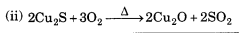# Chemical Reactions and Equations Class 10 Extra Questions with Answers Science Chapter 1

In this page, you can find CBSE Class 10 Science Chapter 1 Extra Questions and Answers Chemical Reactions and Equations Pdf free download, NCERT Extra Questions for Class 10 Science will make your practice complete.

## Class 10 Science Chapter 1 Extra Questions and Answers Chemical Reactions and Equations

Extra Questions for Class 10 Science Chapter 1 Chemical Reactions and Equations with Answers Solutions

### Chemical Reactions and Equations Extra Questions Very Short Answer Type

Question 1.
Why is the combustion of Liquified Petroleum Gas (LPG) a chemical change?
Combustion of Liquified Petroleum Gas (LPG) is a chemical change because, after its combustion, a new substance is formed and cannot be turned back into LPG.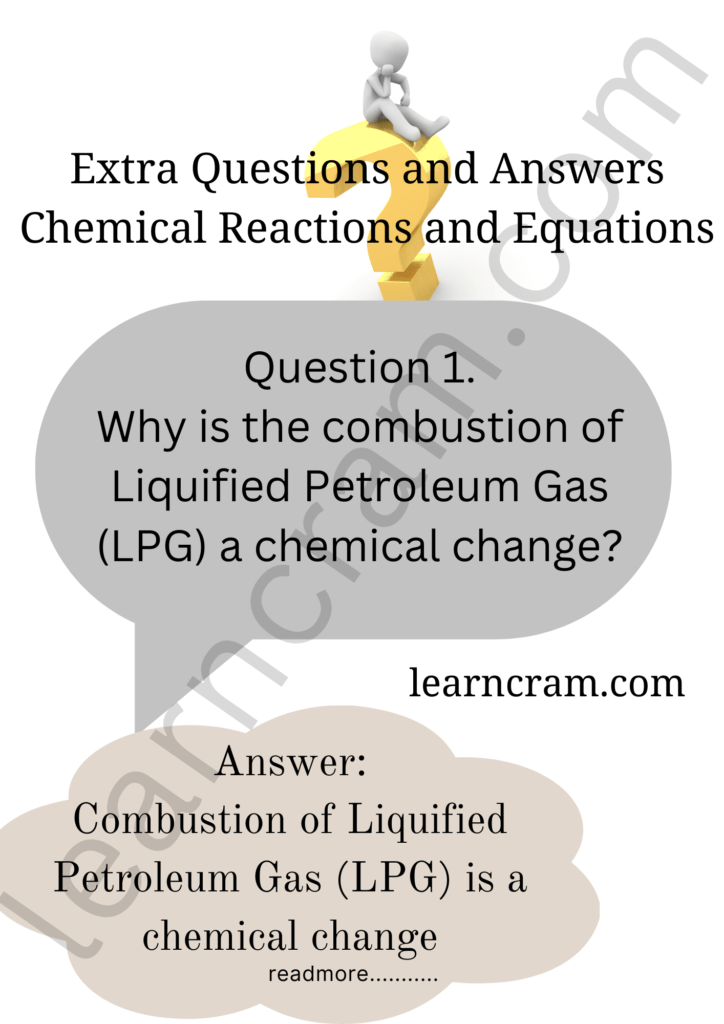Question 2.
What is wrong with the following equation?
Mg + O ➝ MgO
Identify the mistake and balance the equation.
In this equation, oxygen should be in molecular form (O2).
2Mg + O2 ➝ 2MgO.

Question 3.
What is meant by the skeletal equation?
The equation where the number of atoms of each element on both sides of a chemical equation is not equal is called a skeletal equation.Question 4.
Potassium chlorate (KClO3) on heating forms potassium chloride and oxygen. Write a balanced equation for these reactions
2KClO3(s) → 2KCI(s) + 3O2(g)

Question 5.
What do you observe when magnesium ribbon is burnt?
When magnesium ribbon is burnt, formation of white powder is observed along with white dazzling flame.

Question 6.
On heating blue coloured powder of copper (II) nitrate in a boiling tube, copper oxide (black), oxygen gas and a brown gas ‘X’ is formed. Identify the brown gas ‘X’.‘X’ is nitrogen dioxide gas.

Question 7.
Convey the following information in the form of a balanced chemical equation:
“An aqueous solution of ferrous sulphate reacts with an aqueous solution of sodium hydroxide to form a precipitate of ferrous hydroxide and sodium sulphate remains in solution.”
FeSO4(aq) + 2NaOH (aq) → Fe(OH)2(s) + Na2SO4(aq)

Question 8.
Balance the following chemical equation :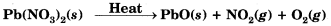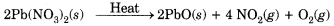Question 9.
Give one example of a combination reaction which is also exothermic.
When quicklime or calcium oxide (Cao) reacts with water, slaked lime (Ca(OH)2] is formed. During this reaction a large amount of heat is released. So, this reaction is an exothermic reaction.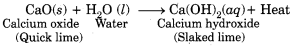Question 10.
Why will the colour of heated copper powder become black when air is passed over it?
When copper powder is heated in the presence of air, black copper oxide is formed.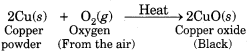Question 11.
What is the difference between the following two types of reactions ?
AgNO3 + HCl → AgCl + HNO3
Mg + 2HCl → MgCl2 + H2
The first reaction is a double displacement reaction whereas second reaction is a single displacement reaction.

Question 12.
Why is hydrogen peroxide kept in coloured bottles ?
This is done in order to cut off light because hydrogen peroxide decomposes into water and oxygen in the presence of light.Question 13.
Consider the following reactions :
(i) Fe + CuSO4 → FeSO4 + Cu
(ii) Cu + FeSO4 → CuSO4 + Fe
Which of these two reactions will take place and why?
Reaction (i) will take place. This is because Fe (iron) is more reactive than Cu (copper) and so it can displace Cu from its compound (CuSO4). On the other hand, copper (Cu) cannot displace iron (Fe), so reaction,
(ii) will not take place.

Question 14.
Give one example of a reaction which is a double displacement reaction as well as a precipitation reaction.Question 15.
Why is photosynthesis considered an endothermic reaction ?
Photosynthesis is an endothermic reaction because energy, in the form of sunlight is absorbed during the process of photosynthesis by green plants.

Question 16.
What type of reaction is represented by the digestion of food in our body?
Decomposition reaction.

Question 17.
How will you test for the gas which is liberated when hydrochloric acid reacts with an active metal ?
When an active metal like Zn reacts with HCl, the gas produced burns with a pop sound which indicates that it is hydrogen gas.
Zn(s) + 2HCl (aq) → ZnCl2(s) + H2 (g)

Question 18.
Can a double displacement reaction take place when the products are highly soluble or highly ionised?
No, double displacement reaction takes place when there is a formation of a slightly soluble salt.

Question 19.
What changes in the colour of iron nails and copper sulphate solution do you observe after keeping the iron nails dipped in copper sulphate solution for about 3O minutes ?
Iron nails become brownish in colour and the blue colour of copper sulphate solution fades. This is because iron displaces copper from copper sulphate solution and forms ferrous sulphate which is light green in colour.

Question 20.
Name the oxidising and reducing agent in the following reaction :
2H2S + SO2 ➝ 2H2O + 3S ↓.
H2S is the reducing agent while SO, is the oxidising agent.

Question 21.
Explain with reason as to why the blue colour of aqueous copper sulphate solution fades away slowly when an iron rod is dipped into it.
Iron being more reactive than copper displaces copper from copper sulphate solution. Thus, blue colour of the solution fades and copper metal gets deposited.Question 22.
Explain redox reaction with example.
Redox Reaction: Those reactions in which oxidation and reduction take place simul taneously, are called redox reactons. Exp.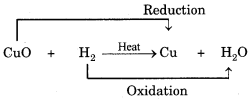### Chemical Reactions and Equations Extra Questions Short Answer Type

Question 1.
Which among the following are physical or chemical changes ?
(a) Evaporation of petrol
(b) Burning of Liquefied Petroleum Gas (LPG)
(c) Heating of an iron rod to red hot
(d) Curdling of milk
(e) Sublimation of solid ammonium chloride
(a) Physical change
(b) Chemical change
(c) Physical change
(d) Chemical change
(e) Physical change.

Question 2.
How do we come to know that a chemical reaction has taken place ?
The presence of any of the following changes helps us to determine that a chemical reaction has taken place.
(i) Formation of new substance(s)
(ii) Change in state
(iii) Change in colour
(iv) Change in temperature
(v) Formation of a precipitate
(vi) Evolution of a gas For example, if on mixing two substances a gas is evolved, then we can say that a chemical reaction has taken place.

Question 3.
Which among the following changes are exothermic or endothermic in nature ?
(a) Decomposition of ferrous sulphate :
(b) Dilution of sulphuric acid
(c) Dissolution of sodium hydroxide in water
(d) Dissolution of ammonium chloride in water
(a) is endothermic as heat is absorbed in these changes.
(b) is exothermic as heat is released in these changes.
(c) is exothermic as heat is released in these changes.
(d) is endothermic as heat is absorbed in these changes.

Question 4.
What is an oxidation reaction ? Give an example of oxidation reaction. Is oxidation an exothermic or an endothermic reaction ?
The reaction in which oxygen combines with other elements or compounds is known as an oxidation reaction. For example, burning of hydrogen is an oxidation process in which hydrogen combines with oxygen to form water.
2H2(g) + O2 (g) ➝ 2H2O(l)
Oxidation reactions are exothermic.

Question 5.
Why do fire flies glow at night?
Fire flies have a protein which in the presence of enzyme undergoes aerial oxidation. This is a chemical reaction which involves emission of visible light. Therefore, fire flies glow at night.

Question 6.
Give reasons :
(a) Aluminium is a reactive metal but is still used for packing food articles.
(b) Red litmus paper turns blue when touched with aqueous solution of magnesium oxide.
(a) On exposure to air, aluminium forms a hard protective layer of aluminium oxide (AI2O3) which prevent further oxidation.
(b) Magnesium oxide is an oxide of a metal, so, it is basic in nature. Due to its basic character it turns red litmus paper blue when touched with its aqueous solution.

Question 7.
Why do we store silver chloride in dark coloured bottles ?
Silver chloride on exposure to sunlight may decompose as per the following reaction.
2AgCl(s) ➝ 2Ag(s) + Cl2(g)
Therefore, it is stored in dark coloured bottles.

Question 8.
Giving an example, list two informations which make a chemical reaction more useful ?
(i) Indicating physical state of reactants and products.
(ii) Indicating energy change in terms of heat.Question 9.
Complete the missing components/variables given as x and y in the following reactions :
(i) Pb(NO2)2(aq) + 2KI (aq) → PbI2(x) + 2KNO2(y)
(ii) Cu(s) + 2AgNO3 (aq) +Cu(NO3)2(aq) + x (s)
(iii) Zn(s) + H2SO4 (aq) + ZnSO4(x) + H2 (y)
(iv) CaCO3(s) → CaO (s) + CO2(g)
(i) x – (s); y – (aq)
(ii) x – 2Ag
(iii) x – (aq); y – (g)
(iv) x – Heat

Question 10.
Zinc liberates hydrogen gas when reacted with dilute hydrochloric acid, whereas copper does not. Explain why.
Zinc is above hydrogen whereas copper is below hydrogen in the activity series of metals. That is why zinc displaces hydrogen from dilute hydrochloric acid, while copper does not.
Zn + HCl → ZnCl2 + H2
Cu + HCl → No reaction

Question 11.
On adding dilute HCl to copper oxide powder, the soluton formed is blue green. Predict the new compound formed which imparts a blue-green colour to the solution.
The new compound formed is copper (II) chloride (CuCl2), which imparts blue-green colour to the solution.Question 12.
A solution of potassium chloride when mixed with silver nitrate solution, an insoluble white substance is formed. Write the chemical reaction involved and also mention the type of the chemical reaction.
KCl(aq) + AgNO3(aq) → AgCl(s) + KNO3(aq)
It is a double displacement and precipitation reaction.

Question 13.
Ferrous sulphate decomposes with the evolution of a gas having a. characteristic odour of burning sulphur. Write the chemical reaction involved and identify the type of reaction.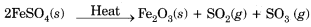It is a thermal decomposition reaction.

Question 14.
Identify the substance oxidised, substance reduced, oxidising agent and reducing agent:
MnO2 + 4HCl → MnCl2 + 2H2O + Cl2
Substance oxidised : HCl
Substance reduced : MnO2
Oxidising agent : MnO2
Reducing agent : HCl

Question 15.
Grapes hanging on the plant do not ferment but after being plucked from the plant can be fermented. Under what conditions do these grapes ferment? Is it a chemical or a physical change ?
Grapes when attached to the plants are living and therefore, their own immune system prevents fermentation. The microbes can grow in the plucked grapes and under anaerobic conditions these can be fermented. This is a chemical change.

Question 16.
A copper coin was kept dipped in silver nitrate solution for a few hours/ days. What will happen to the copper coin ? What will happen to the colour of the solution ?
Copper is more reactive than silver. Hence, it displaces silver from the silver nitrate solution according to the given reaction.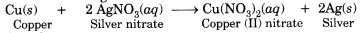The silver thus formed is deposited on the surface of copper, thereby giving it a white shining appearance.
The solution becomes blue due to the formation of copper nitrate..

Question 17.
Identify the reducing agent in the following reactions.
(a) 4NH3 + 5O2 → 4NO + 6H2O
(b) H2O + F2 → HF + HOF
(c) Fe2O3 + 3CO → 2Fe + 3CO2
(d) 2H2 + O2 → 2H2O
(a) Ammonia (NH3)
(b) Water (H2O) as F2 is getting reduced to HF
(c) Carbon monoxide (CO)
(d) Hydrogen (H2)

Question 18.
What is the role of a catalyst in a chemical reaction ?
Catalyst changes (usually increases but sometimes decreases) the rate of a chemical reaction without itself being consumed in the reaction.

Question 19.
Translate the following statements into chemical equations and balance them:
(i) Lead nitrate reacts with sulphuric acid to form a precipitate of lead sulphate and nitric acid. :
(ii) Magnesium burns in the presence of nitrogen to form magnesium nitride.
(iii) Aluminium metal strip is added in hydrochloric acid to produce aluminium chloride and hydrogen gas.
(i) Pb(NO3)2(aq) + H2SO4 (aq) ➝ PbSO4(s) + 2HNO3 (aq)
(ii) 3Mg(s) + N2 (g) ∆ ➝ Mg3 N2(s)
(iii) 2Al(s) + 6HCl (aq) + 2AlCl3(aq) ➝ 3H2(g)

Question 20.
Write the balanced chemical equations for the following reactions :
(a) Sodium carbonate on reaction with hydrochloric acid in equal molar concentrations gives sodium chloride and sodium hydrogen carbonate.
(b) Sodium hydrogencarbonate on reaction with hydrochloric acid gives sodium chloride, water and liberates carbon dioxide.
(c) Copper sulphate on treatment with potassium iodide precipitates cuprous iodide (Cu2I)2 liberates iodine gas and also forms potassium sulphate.
(a) Na2CO3 + HCl → NaCl + NaHCO3
(b) NaHCO3 + HCl + NaCl + H2O + CO2
(c) 2CusO4 + 4KI → Cu2l2 + 2K2SO4 + l2

Question 21.
Write the balanced chemical equations for the following reactions and identify the type of reaction in each case.
(i) Nitrogen gas is treated with hydrogen gas in the presence of a catalyst at 773 K to form ammonia gas.
(ii) Sodium hydroxide solution is treated with acetic acid to form sodium acetate and water.
(iii) Ethanol is warmed with ethanoic acid to form ethyl acetate in the presence of concentrated H2SO4.
(iv) Ethene is burnt in the presence of oxygen to form carbon dioxide, water and releases heat and light.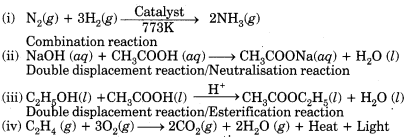Redox reaction/Combustion reaction

Question 22.
Write the balanced chemical equations for the following reactions and identify the type of reaction in each case.
(a) Thermit reaction, iron (III) oxide reacts with aluminium and gives molten iron and aluminium oxide.
(b) Magnesium ribbon is burnt in an atmosphere of nitrogen gas to form solid magnesium nitride.
(c) Chlorine gas is passed in an aqueous potassium iodide solution to form potassium chloride solution and solid iodine.
(d) Ethanol is burnt in air to form carbon dioxide, water and releases heat.
(a) Fe2O3(s) + 2Al(s) + AI2O3(s) + 2Fe(l) + Heat Displacement reaction
(b) 3Mg(s) + N2 (g) → Mg3 N2(s) Combination reaction
(c) 2KI(aq) + CI2(g) → 2Kc1(aq) + I2(s) Displacement reaction
(d) C2H5OH(I) + 3O2(g) → 2CO2(g) + 3H2O(I) + Heat Combustion reaction

Question 23.
Name the type of chemical reaction represented by the following equation:
(i) CaO + H2O → Ca(OH)2
(ii) 3BaCl, + AI2(SO2) → 2AICI3 + 3BaSO4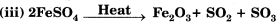(i) Combination reaction.
(ii) Double displacement reaction.
(iii) Thermal decomposition reaction.

Question 24.
Translate the following statements into chemical equations and then balance the equations :
(i) Phosphorus burns in oxygen to give phosphorus pentoxide.
(ii) Aluminium metal replaces iron from ferric oxide, Fe2O3, giving aluminium oxide and iron.
(iii) Carbon disulphide burns in air to give carbon dioxide and sulphur dioxide.
(iv) Barium chloride reacts with zinc sulphate to give zinc chloride and barium sulphate.
(i) P4 + 5O2 → 2P2O5
(ii) 2Al + Fe2O3 → AI2O3 + 2Fe
(iii) CS2 + 3O2 → CO2 + 2SO2
(iv) BaČl2 + ZnSO2 → ZnCl2 + BaSO4

Question 25.
(i) What is observed when a solution of potassium iodide is added to a solution of lead nitrate taken in a test tube ?
(ii) What type of reaction is this?
(iii) Write a balanced chemical equation to represent the above reaction.
(i) A yellow precipitate of lead iodide (PbI,) is formed.
(ii) Precipitation reaction/Double displacement reaction.Question 26.
(i) What happens when silver nitrate solution is added to sodium chloride solution ? Write the equation for the reaction which takes place.
(ii) Name the type of reaction involved.
(i) When silver nitrate solution is added to sodium chloride solution, a white precipitate of silver chloride is formed along with sodium nitrate solution.(ii) This is double displacement reaction and precipitation reaction.

Question 27.
(i) What is the colour of ferrous sulphate crystals ? How does this colour change after heating ?
(ii) Name the products formed on strongly heating ferrous sulphate crystals. What type of chemical reaction occurs in this change?
(i) The colour of ferrous sulphate crystals is green. On heating, FeSO47H2O first decomposes to form anhydrous ferrous sulphate (FeSO) which is white in colour.
(ii) The products formed on strongly heating ferrous sulphate crystals are ferric oxide, sulphur dioxide and sulphur trioxide.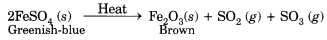This is a type of thermal decomposition reaction.

Question 28.
Identify the oxidising agent (oxidant) in the following reactions.
(i) Pb3O4 + 8HCl → 3PbCl2 + Cl2 + 4H2O
(ii) 2Mg + O2 → 2Mgo
(iii) CuSo4 + Zn → Cu + ZnSO4
(iv) V2O5 + 5Ca → 2V + 5Cao
(v) 3fe + 4H2O → Fe3O4 + 4H2
(vi) CuO + H2 → Cu + H2O
(i) Pb3O4
(ii) O2
(iii) CuSO4
(iv) V2O2
(v) H2O
(vi) Cuo

Question 29.
Solid calcium oxide was taken in a container and water was added slowly to it
(i) State the two observations made in the experiment.
(ii) Write the name and chemical formula of the product formed.
(i) Following are the two observations :
(a) Calcium oxide (Cao) reacts vigorously with water to form slaked lime.
(b) The container becomes hot because a large amount of heat is released during this reaction.
(ii) The product formed is slaked lime for which the chemical formula is Ca(OH)2.Question 30.
A substance X, which is an oxide of a group 2 element, is used intensively in the cement industry. This element is present in bones also. On treatment with water it forms a solution which turns red litmus blue. Identify X and also write the chemical reactions involved.
X is calcium oxide, also called quick lime. It reacts with water to form calcium hydroxide which is basic in nature. So, it turns red litmus blue.
CaO(s) + H2O (l) + Ca(OH2)(aq)

Question 31.
A magnesium ribbon is burnt in oxygen to give a white compound X accompanied by emission of light. If the burning ribbon is now placed in an atmosphere of nitrogen, it continues to burn and forms a compound Y.
(i) Write the chemical formulae of X and Y.
(ii) Write the balanced chemical equation when X is dissolved in water.
2Mg + O2 → 2MgO + Light
3Mg + N2 → Mg Ni
(i) X is MgO; Y is Mg3 N2
(ii) MgO + H2O → Mg(OH)2

Question 32.
Identify the type of reaction in the following examples :
(i) Na2 SO4 (aq) + BaCl2 (aq) → BaSO4(s) + NaCl (aq)
(ii) Fe (8) + CuSO4 (aq) → FeSO4 (aq) + Cu (s)
(iii) 2H2 (g) + O2(g) 2H2O(l)
(i) Double displacement reaction,
(ii) Displacement reaction,
(iii) Combination reaction.

Question 33.
During the reaction of some metals with dilute hydrochloric acid, following observations were made :
(i) Silver metal does not show any change.
(ii) The temperature of the reaction mixture rises when aluminium (Al) is added.
(iii) The reaction of sodium metal is found to be highly explosive.
(iv) Some bubbles of a gas are seen when lead (Pb) is reacted with the acid.
Explain these observations giving suitable reasons.
(i) Silver being a less reactive metal than hydrogen does not displace hydrogen from HCI. Hence, it does not react with dilute HCI.
(ii) The temperature of the reaction mixture rises when aluminium is added because it is an exothermic reaction.
(iii) Reaction of sodium metal is found to be highly explosive because it is an exothermic reaction.
(iv) When lead is treated with hydrochloric acid, bubbles of hydrogen gas are evolved.
Pb(s) + 2HCl (aq) → PbCl2(aq) + H2(g)

Question 34.
A silver article generally turns black when kept in the open for a few days. The article when rubbed with toothpaste again stars shining.
(a) Why do silver articles turn black when kept in the open for a few days ? Name the phenomenon involved.
(b) Name the black substance formed and give its chemical formula. .
(a) Metals such as silver when attacked by substances around it such as moisture, acids, gases etc., are said to corrode and this phenomenon is called corrosion.
(b) The black substance is formed because silver (Ag) reacts with H2S present in air. It forms thin black coating of silver sulphide (Ag2S).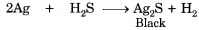### Chemical Reactions and Equations Extra Questions Long Answer Type

Question 1.
Consider the chemical equation given below and answer the questions that follow: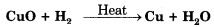(i) Name the substance which is getting oxidised.
(ii) Name the substance which is getting reduced.
(iii) Name the oxidising agent.
(iv) Name the reducing agent.
(v) What type of a reaction does this equation represent ?
(i) The substance getting oxidised is H2
(ii) The substance getting reduced is CuO.
(iii) CuO is the oxidising agent.
(iv) H2 is the reducing agent.
(v) Since oxidation and reduction is taking place simultaneously, this reaction is an example of redox reaction.

Question 2.
Give the characteristic tests for the following gases:
(i) CO2
(ii) SO2
(iii) O2
(iv) H2
The characteristic test for
(i) Carbon dioxide (CO2) gas turns lime water milky when passed through it due to the formation of insoluble calcium carbonate.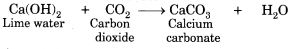When CO2 gas is passed in excess through lime water, milkiness disappears due to formation of soluble calcium hydrogen carbonate.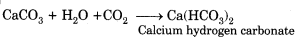(ii) Sulphur dioxide (SO2) gas when passed through acidic potassium permanganate solution (purple in colour) turns it colourless because SO2 is a strong reducing agent.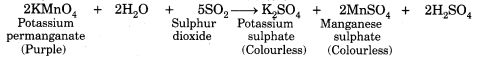(iii) The evolution of oxygen (O2) gas during a reaction can be confirmed by bringing a burning candle near the mouth of the test tube containing the reaction mixture. The intensity of the flame increases because oxygen supports burning.
(iv) Hydrogen (H2 gas burns with a pop sound when a burning candle is brought near it.

Question 3.
With the help of an activity explain that hydrogen and oxygen are released when an electric current is passed through water.(i) Take a plastic vessel. Drill two holes at its bottom and set rubber stoppers in these holes.
(ii) Insert carbon electrodes in these rubber Plastic mug stoppers and connect these electrodes to a 6 volt battery and a switch.
(iii) Fill the vessel with water such that the electrodes are immersed. Add a few drops Test tube wenn of dilute sulphuric acid to the water in the nows Water vessel.
(iv) Take two graduated test tubes filled Water with water and invert them over the two carbon electrodes.
(v) Switch on the current.
(vi) After sometime, you will observe the formation of bubbles at both the electrodes. These bubbles displace water in the graduated tubes.
(vii) Once the test tubes are filled with the respective gases, remove them carefully.
(viii) Test these gases one by one by bringing a burning splinter of wood close to the mouth of test tubes.

When the glowing splinter of wood is brought close to the mouth of one test tube, it relights and when it is brought close to the mouth of other test tube, the gas burns with a pop. Oxygen is the only common gas that relights the splinter and hydrogen gas burns with a pop.

Question 4.
What happens when a piece of:
(a) zinc metal is added to copper sulphate solution ?
(b) aluminium metal is added to dilute hydrochloric acid ?
(c) silver metal is added to copper sulphate solution ? Also, write the balanced chemical equation if the reaction occurs.
(a) Zinc being more reactive than copper displaces copper from its solution and a solution of zinc sulphate is obtained.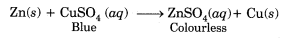(b) Aluminium being more reactive displaces hydrogen from dilute hydrochloric acid solution and hydrogen gas is evolved.(c) Silver metal being less reactive than copper cannot displace copper from its salt solution. Therefore, no reaction occurs.
Ag(s) + CuSO4 (aq) → No reaction

Question 5.
What happens when zinc granules are treated with dilute solution of H2SO4, HCI, HNO2, NaCl and NaOH ? Also write the chemical equations if reaction occurs.
The reaction of Zn granules with
(i) dilute H2SO4
Zn(s) + H2SO4 (aq) → ZnSO4(aq) + H2(g)
(ii) dilute HCI
Zn(s) + 2HCl (aq) → ZnCl2(aq) + H2 (g)
(iii) dilute HNO,
Reaction with dilute HNO, is different as compared to other acids because nitric acid is an oxidising agent and it oxidises H2 gas evolved to H2O.
4Zn(s) + 10HNO2 (aq) + 4Zn(NO3)2(aq) + 5H2O (l) +N2O (g)
(iv) NaCl solution
Zn(s) + NaCl(aq) → No reaction
(v) NaOH solution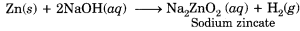Question 6.
What happens when : (Give chemical reactions only)
(i) Ferrous sulphate is heated.
(ii) Sodium metal reacts with water.